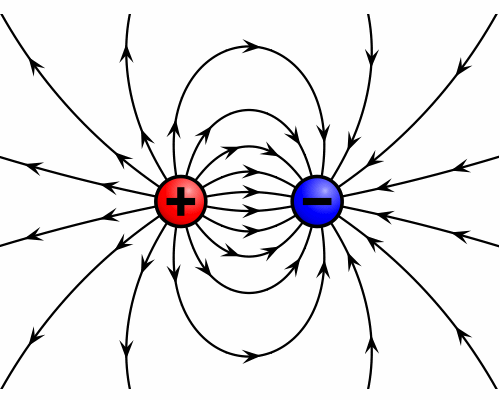Skip to main content

# 1. Charge Carriers and the Electric Field

To discuss electricity, we must introduce the concept of charged particles. Fundamental particles such as electrons have associated with them a specific electric charge, or intrinsic property that determines the Coulomb forces or electromagnetic force they exhibit on each other. Electrons have a charge commonly denoted as –e, or -1.602 x 10-19 Coulombs (C). The charge of a proton is e, or the same number but positive, while a neutron has no charge. Later we will discuss the hole, which is a vacancy left in an orbital when an electron jumps to a higher energy state. Interestingly, even holes are treated as charge carriers in solar physics, and they possess a positive charge.

Some points about charged particles:

• Opposite charges (like a proton and electron) attract, while like charges repel.
• The magnitude of the electric forces that particles exert on each other depends on the distance. The force, like gravity, is inversely proportional to the square of the distance. This means that the further apart two particles are, the less force they exert on each other.
• Particles create an electric field, even with no other particles around. A proton’s electric field is directed outward, while an electron’s field is directed inward. These fields can be thought of as a particle warping space around it, and this field then interacts with other particles. Thus, the forces between particles are a result of the electric fields created by all the particles individually.Two oppositely charged particles and their electric fields (with arrows pointing in the direction of the force); the proton is left and the electron is right.

Source: Wikipedia.org. 27 Feb. 2012.

• Was this article helpful?